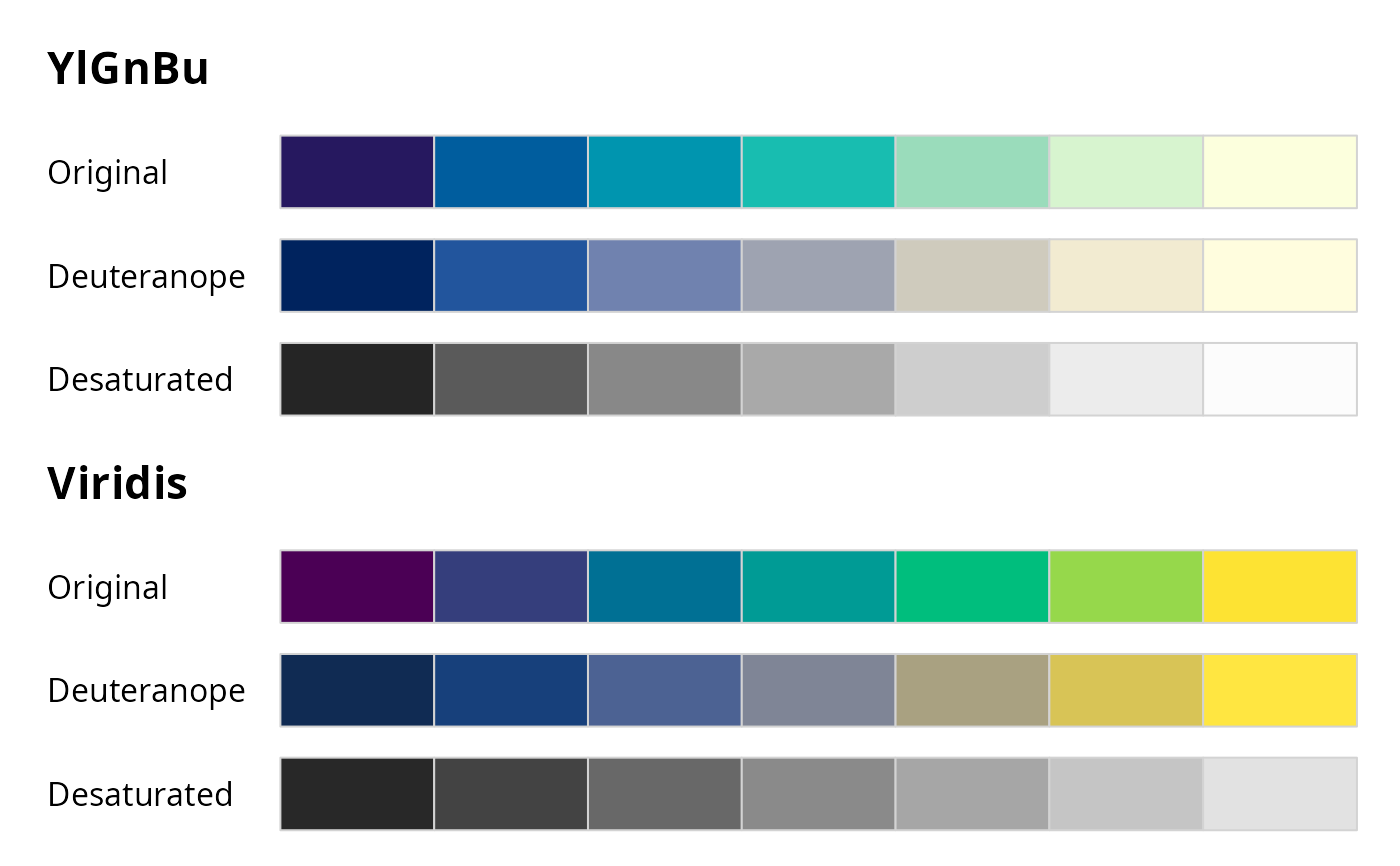Visualization of color palettes in columns of color swatches.

swatchplot(
x,
...,
nrow = 20,
border = NULL,
sborder = NULL,
off = NULL,
mar = NULL,
line = NULL,
cex = NULL,
font = 1:2,
cvd = FALSE
)

## Arguments

x

character vector/matrix (or list of character vectors/matrices) containing color hex codes.

...

further (possibly named) character vectors/matrices with color hex codes.

nrow

integer specifying the maximal number of rows of swatches. (The actual number might be lower in order to balance the rows used in each column.)

border

color for border of individual color rectangles. By default "lightgray" for up to 9 colors, "transparent" otherwise.

sborder

color for border of the entire palette swatch. By default "lightgray" if border is "transparent" and "lightgray" otherwise (if off = 0).

off

numeric vector of length 2. Offset in horizontal and vertical direction (specified as a fraction of the rectangle for one color). By default, the horizontal offset is 0.3 for up to 5 colors and 0 otherwise, and the vertical offset is 0.1.

mar

numeric vector of length 4, specifying the margins of column of color swatches.

line

numeric. Line in which the palette names (if any) are printed in the margin.

cex, font

numeric vectors of length 1 or 2. Specifications for the annotation text for the individual palettes and lists of palettes, respectively.

cvd

logical or character indicating whether color vision deficiencies should be emulated with desaturate, deutan, protan, tritan.

## Value

swatchplot invisibly returns a matrix with colors and annotations.

## Details

The function swatchplot is a convenience function for displaying collections of palettes that can be specified as lists or matrices of character color specifications. Essentially, the function just calls rect but the value-added are the heuristics used for choosing default labels, margins, spacings, borders. These are selected to work well for hcl_palettes and might need further tweaking in future versions.

## Examples

## swatches of several palette vectors
swatchplot(
"Hue"       = sequential_hcl(5, h = c(0, 300), c = c(60, 60), l = 65),
"Chroma"    = sequential_hcl(5, h = 0, c = c(100, 0), l = 65, rev = TRUE, power = 1),
"Luminance" = sequential_hcl(5, h = 260, c = c(25, 25), l = c(25, 90), rev = TRUE, power = 1),
off = 0
)## swatches of named palette matrices
bprg <- c("Blues", "Purples", "Reds", "Greens")
swatchplot(
"Single-hue"            = t(sapply(paste(bprg, 2), sequential_hcl, n = 7)),
"Single-hue (advanced)" = t(sapply(paste(bprg, 3), sequential_hcl, n = 7)),
"Multi-hue (advanced)"  = t(sapply(bprg,           sequential_hcl, n = 7)),
nrow = 5
)## swatches with color vision deficiency emulation
swatchplot(sequential_hcl(7, "Viridis"), cvd = TRUE)swatchplot(
"YlGnBu"  = sequential_hcl(7, "YlGnBu"),
"Viridis" = sequential_hcl(7, "Viridis"),
cvd = c("deutan", "desaturate")
)